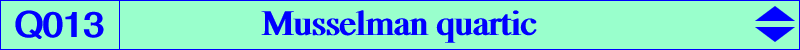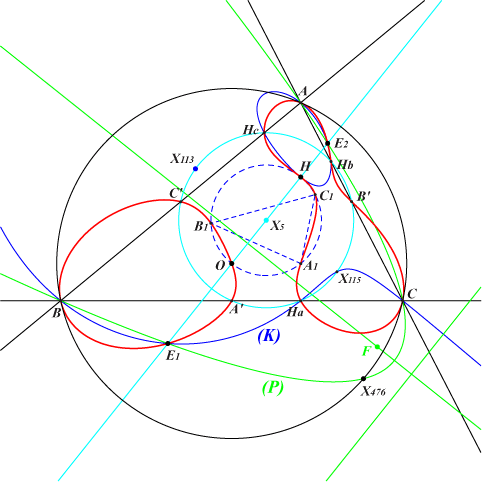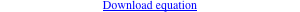too complicated to be written here. Click on the link to download a text file.X(3), X(4) A', B', C' : midpoints Ha, Hb, Hc : feet of the altitudes A1, B1, C1 : projections of O on the altitudes E1, E2 see belowDenote by A1, B1, C1 the reflections of P in A, B, C and by A3, B3, C3 the reflections of H in the lines AP, BP, CP. The three circles AB1C1, BC1A1, CA1B1 have a common point D on the circumcircle. The three circles PAA3, PBB3, PCC3 have P in common and another point L also on the circumcircle. These points D, L are antipodes if and only if P lies on the Thomson cubic (Musselman, Some loci connected with a triangle. Monthly, p.354-361, June-July 1940). On the other hand, they coincide if and only if P lies on the bicicular quartic Q013 we call the Musselman quartic. Q013 is also the locus of M whose orthotransversal and polar in the circumcircle are perpendicular. When these are parallel, we find the Orthocubic K006. Q013 is a bicircular quartic which is tritangent at A, B, C to the circumcircle (O). It is also bitangent to the line at infinity at the circular points. Q013 has the following properties : it contains O with a tangent passing through X(924). it containts H with a tangent perpendicular to the Euler line. it meets the Euler line again at two other points E1, E2 which are its intersections with the circum-parabola (P) with perspector X(115). The orthotransversals of these two points coincide and they are parallel at X(113) to the Euler line. (P) is the isogonal transform of the tangent at X(110) to (O). (P) also contains X(476), X(523), X(685), X(850), X(892), X(2395), X(2501). E1, E2 also lie on the circular cubic (K) = pK(X2501, X4). See CL019. Hence E1, E2 are conjugated under the isoconjugation with pole X(2501). it has 8 known points in common with the nine-point circle : the midpoints of ABC, the feet of the altitudes, the circular points at infinity. it is a member of the pencil of bicircular quartics generated by the union of the circum-circle and the nine-point circle on the one hand, the union of the sidelines of ABC and the line at infinity on the other hand.